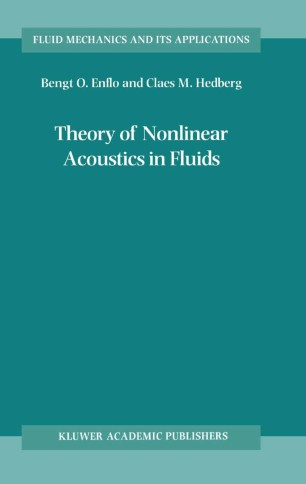# Theory of Nonlinear Acoustics in Fluids

• Bengt O. Enflo
• Claes M. HedbergBook

Part of the Fluid Mechanics and Its Applications book series (FMIA, volume 67)

1. Front Matter
Pages i-xiii
2. Pages 1-10
3. Pages 11-29
4. Pages 31-51
5. Pages 93-147
6. Pages 149-197
7. Pages 199-218
8. Pages 219-250
9. Back Matter
Pages 251-282

### Introduction

The aim of the present book is to present theoretical nonlinear aco- tics with equal stress on physical and mathematical foundations. We have attempted explicit and detailed accounting for the physical p- nomena treated in the book, as well as their modelling, and the f- mulation and solution of the mathematical models. The nonlinear acoustic phenomena described in the book are chosen to give phy- cally interesting illustrations of the mathematical theory. As active researchers in the mathematical theory of nonlinear acoustics we have found that there is a need for a coherent account of this theory from a unified point of view, covering both the phenomena studied and mathematical techniques developed in the last few decades. The most ambitious existing book on the subject of theoretical nonlinear acoustics is ”Theoretical Foundations of Nonlinear Aco- tics” by O. V. Rudenko and S. I. Soluyan (Plenum, New York, 1977). This book contains a variety of applications mainly described by Bu- ers’ equation or its generalizations. Still adhering to the subject - scribed in the title of the book of Rudenko and Soluyan, we attempt to include applications and techniques developed after the appearance of, or not included in, this book. Examples of such applications are resonators, shockwaves from supersonic projectiles and travelling of multifrequency waves. Examples of such techniques are derivation of exact solutions of Burgers’ equation, travelling wave solutions of Bu- ers’ equation in non-planar geometries and analytical techniques for the nonlinear acoustic beam (KZK) equation.

### Keywords

Diffusion fluid mechanics mechanics stress thermodynamics wave wave equation

#### Authors and affiliations

• Bengt O. Enflo
• 1
• Claes M. Hedberg
• 2
1. 1.Department of MechanicsKungl. Tekniska HögskolanStockholmSweden
2. 2.Department of Mechanical EngineeringBlekinge Tekniska HögskolaKarlskronaSweden

### Bibliographic information

• DOI https://doi.org/10.1007/0-306-48419-6